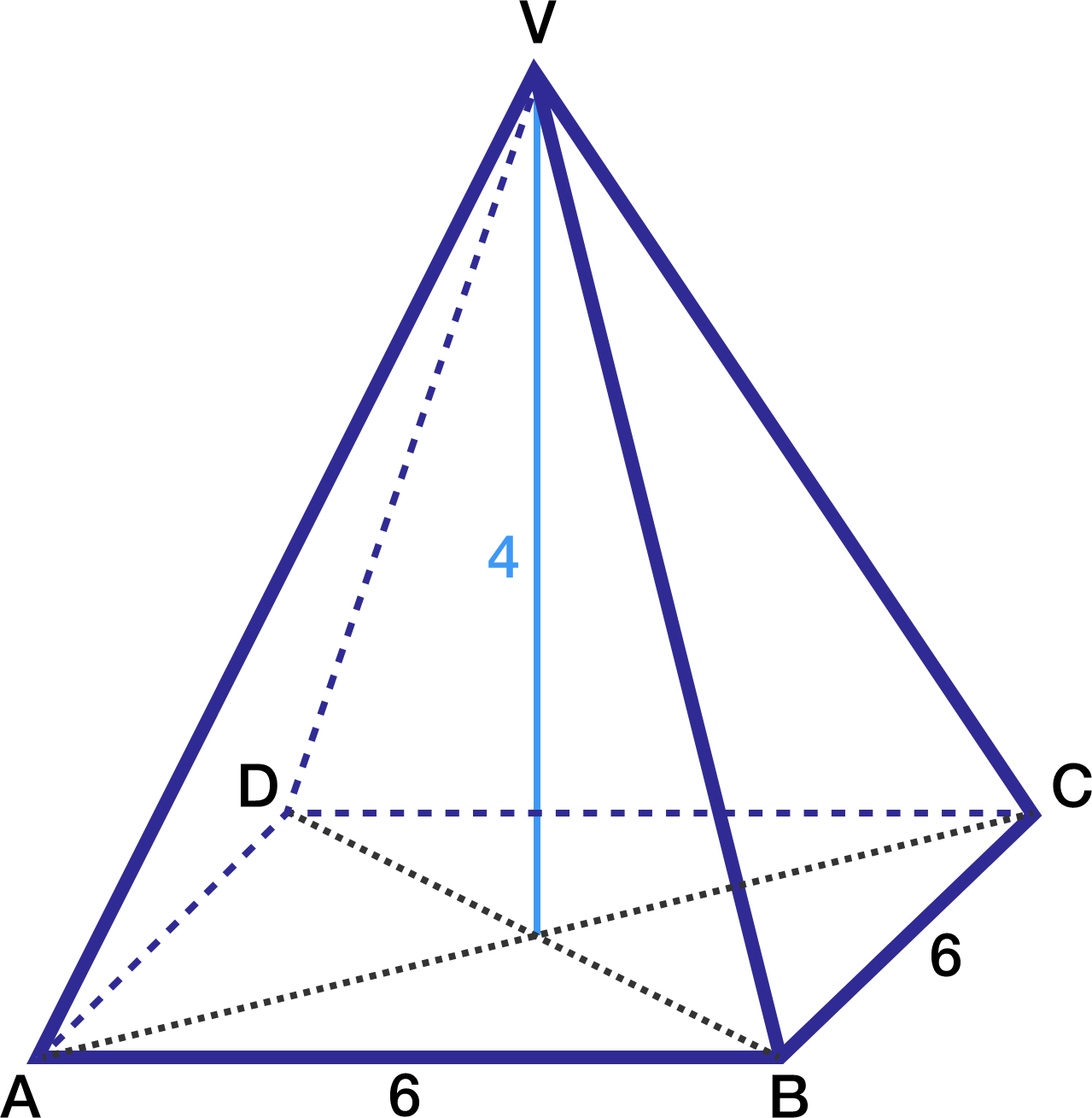# Sides Of A Square Pyramid

Geometry Level 1Consider a pyramid with a square base of side length 6 m. The sides of the pyramid are identical isosceles triangles that meet at a common point directly above the center of the base. This point is exactly 4 m above the center.

The area of the square base is clearly $6 \times 6 = 36\text{ m}^2$. What is the total area of the 4 triangular sides, in square meters?

×